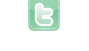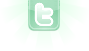Report as inappropriate.

# Rigid Body Physics Engine

This is the rewritten, restructured, and reorganized version of my physics engine.

This time I decided to start with circles before I get to polygons.

The collisions do not affect the rotation yet. (But rotation already does affect the collision)

I also created better graphics in GIMP in which you can clearly see the rotation.

The arrow keys control the red ball.

*UPDATE* Proper collisions, but no friction yet

Your browser does not support the canvas tag.
This is looking really great! The collisions seem to be working really well, and running the scenario for a long period of time doesn't seem to yield any problems :) Just out of curiosity, have you seen my Rigid Body physics engine?
Yes, I did see it. I am trying to figure out collisions with friction, now.
I created my own collision algorithm, but I have no idea how to implement rotation it it. norm = norm.unit(); PhysicsObject a = this; Vector2D ua = a.velocity; Vector2D ub = b.velocity; Vector2D uab = ua.subtract(ub); if(uab.dotProduct(norm) < 0) { double e = (a.elasticity + b.elasticity) / 2; double f = Math.min(a.friction, b.friction); Vector2D tang = norm.perpendicular(); Vector2D uaNorm = ua.projectOnto(norm); Vector2D uaTang = ua.projectOnto(tang); Vector2D ubNorm = ub.projectOnto(norm); Vector2D ubTang = ub.projectOnto(tang); Vector2D vaNorm = elasticCollision(uaNorm, a.mass, ubNorm, b.mass, e); Vector2D vbNorm = elasticCollision(ubNorm, b.mass, uaNorm, a.mass, e); Vector2D inelasticVTang = inelasticCollision(uaTang, a.mass, ubTang, b.mass); Vector2D vaTang = inelasticVTang.scale(f).add(uaTang.scale(1-f)); Vector2D vbTang = inelasticVTang.scale(f).add(ubTang.scale(1-f)); a.velocity = vaNorm.add(vaTang); b.velocity = vbNorm.add(vbTang); } I wonder if a solution would be to have a method that applies an impulse to a certain point on the body or something?
I think the impulse idea would work well. We know that since we are avoiding friction for the moment, a collision can only exert force along the normal. (And consequently with spheres, rotation does not occur) If we take into account friction, then there is a force tangential to the surface. Anyway, when you do impulse on a point, a percentage of that force goes into rotation, and the other percentage goes into translation. This is a bit more difficult for me though, since I do my simulating in a completely different matter, by simulating the elasticity and deformation of the object XD
For the impulse function, I guess you can find the normal velocity component and apply that to the object's linear velocity. But for the tangential component, I am not sure how to calculate how much of it will go into rotation and how much will go into translation.
As for now it seems to be working well with my impulse method idea, but no rotation yet...
about the incodent it is OK i have gone through this situation before
I don't understand what this is about and why you posted this in my scenario.
I don't understand what this is about and why you posted this in my scenario.
Oops, I accidentally clicked submit twice.